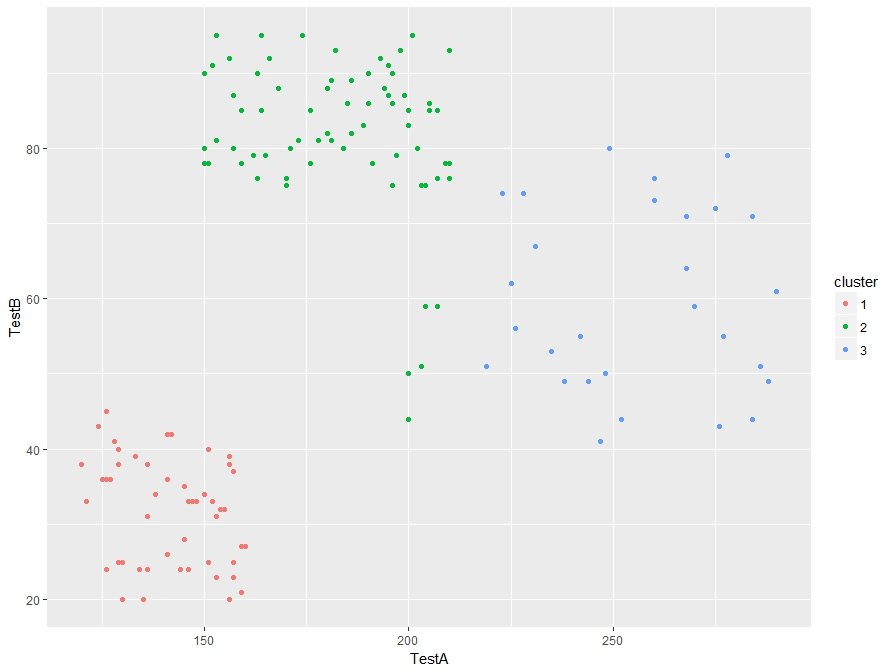# R: K-Means Clustering

Note: This is an introductory lesson with a made up data set. After you are finished with this tutorial, if you want to see a nice real world example, head on over to Michael Grogan’s website:

http://www.michaeljgrogan.com/k-means-clustering-example-stock-returns-dividends/

K Means Cluster will be our introduction to Unsupervised Machine Learning. What is Unsupervised Machine Learning exactly? Well, the simplest explanation I can offer is that unlike supervised where our data set contains a result, unsupervised does not.

Think of a simple regression where I have the square footage and selling prices (result) of 100 houses. Taking that data, I can easily create a prediction model that will predict the selling price of a house based off of square footage. – This is supervised machine learning

Now, take a data set containing 100 houses with the following data: square footage, house style, garage/no garage, but no selling price. We can’t create a prediction model since we have no knowledge of prices, but we can group the houses together based on commonalities. These groupings (clusters) can be used to gain knowledge of your data set.

I think seeing it in action will help.

Here is the data set: cluster

The data we will be looking at test results for 149 students.The task at hand is to group the students into 3 groups based on the test results. Now one thing any teacher will let you know is that some kids perform well in one subject and perhaps not so well in another. So we can’t simply group them on the score performance on one test. And when you are dealing with real world data, you might be looking at 20 -100 test/quiz scores per student.

So what we are going to do is let the computer decide how to group (or cluster) them.

To do so, we are going to be using K-means clustering. K-means clustering works by choosing random points (centroids). It then groups the data points around the centroids based which centroid the points are closest to.

## Let’s get started

```st <- read.csv(file.choose())

our dataNow let’s run the data through a Kmeans() algorithm

First, we are only going to want to focus on columns 2 and 3 in the data set since column 1 (studentID) is basically a label and provides no value in prediction.

To do this, we subset the data: st[,2:3] – which means I want all row ([,) and columns 2-3 (2:3])Now the code to make our clusters

```stCl <- kmeans(st[, 2:3], 3, nstart = 20)
stCl```

The syntax is kmeans(DATA, Number of clusters, Numbers of random starts)

Number of clusters I picked as 3 because I know this works well with the data, picking the right number usually takes a little trial and error in real life

Number of random starts is how many times you want the algorithm to be rerun (choosing new centroids each time) and choosing the result where the clusters are tightest.

Below is the output of our Kmeans – note the cluster means, this tells us the mean score for TestA and TestB set in each cluster.Hey, if you are a math junkie, this may be all you want. But if you are looking for some more practical value here, lets move on.

First, we need to add a column to our data set that shows our columns.

Now since we read our data from a csv, it is a data frame. If you can’t remember that, you can always run the command is.data.frame(st) to test it out.

Do you remember how to add a column to a data frame?

Well, there are multiple ways, but this is, in my opinion, the easiest way.

st\$cluster <- stCl\$cluster

```is.data.frame(st)
st\$cluster <- stCl\$cluster

Here is the resultNow with the clusters, you can group your students based their assigned cluster.

Technically we are done here. We have successfully grouped the students. But what if you want to make sure you did a good job. One quick check is to graph your work.

Before we can graph, we have to make sure our st\$cluster column is set as a factor, then using ggplot, we can graph it. (if you don’t have ggplot2 installed, you will need to run this line: install.packages(“ggplot2”)

```library(ggplot2)
st\$cluster <- as.factor(st\$cluster)
ggplot(st, aes(TestA, TestB, color = cluster)) + geom_point()```

And here is our output. The groups look pretty good.